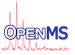OpenMS  2.8.0
PeakIntensityPredictor Class Reference

Predict peak heights of peptides based on Local Linear Map model. More...

`#include <OpenMS/ANALYSIS/PIP/PeakIntensityPredictor.h>`

Collaboration diagram for PeakIntensityPredictor:
[legend]

## Public Member Functions

PeakIntensityPredictor ()
Constructors and Destructors. More...

virtual ~PeakIntensityPredictor ()
destructor More...

double predict (const AASequence &sequence)
Returns predicted peak heights (intensities) of a single peptide. More...

double predict (const AASequence &sequence, std::vector< double > &add_info)
Returns predicted peak heights (intensities) of a single peptide. More...

std::vector< double > predict (const std::vector< AASequence > &sequences)
Returns predicted peak heights (intensities) of several peptides. More...

std::vector< double > predict (const std::vector< AASequence > &sequences, std::vector< std::vector< double > > &add_info)
Returns predicted peak heights (intensities) of several peptides. More...

## Private Member Functions

double map_ (const std::vector< double > &data)
calculate and return predicted value based on given LocalLinearMap model for corresponding aaindex variables More...

Size findWinner_ (const std::vector< double > &data)
find winning prototype More...

std::vector< double > calculateAddInfo_ (const std::vector< double > &data)
calculate assignments of peptides to cluster and the corresponding error More...

std::vector< double > getPropertyVector_ (const AASequence &sequence)
Calculates an array of properties for an amino acid sequence. More...

PeakIntensityPredictor (const PeakIntensityPredictor &llmModel)
copy constructor not implemented => private More...

PeakIntensityPredictoroperator= (const PeakIntensityPredictor &peakIntensityPredictor)
assignment operator not implemented => private More...

## Private Attributes

LocalLinearMap llm_
Local Linear Map model. More...

## Detailed Description

Predict peak heights of peptides based on Local Linear Map model.

This class can be used for predictions of peptide peak heights (referred to as intensities) from a peptide sequence by a Local Linear Map (LLM) model. A general introduction to the Peak Intensity Predictor (PIP) can be found in the PIP Tutorial.

The predictor performs only on the peptides sequences as an AASequence representation. Every sequence is transformed to an 18 dimensional data vector representing certain chemical characteristics and is loaded into the trained LocalLinearMap model to find the predicted peptides peak intensity.

Every predictor object calls the appropriate LocalLinearMap model, transforms the given sequences and creates a vector space in which the LocalLinearMap performs.

## ◆ PeakIntensityPredictor() [1/2]

 PeakIntensityPredictor ( )

Constructors and Destructors.

default constructor

## ◆ ~PeakIntensityPredictor()

 virtual ~PeakIntensityPredictor ( )
virtual

destructor

## ◆ PeakIntensityPredictor() [2/2]

 PeakIntensityPredictor ( const PeakIntensityPredictor & llmModel )
private

copy constructor not implemented => private

## Member Function Documentation

 std::vector calculateAddInfo_ ( const std::vector< double > & data )
private

calculate assignments of peptides to cluster and the corresponding error

## ◆ findWinner_()

 Size findWinner_ ( const std::vector< double > & data )
private

find winning prototype

## ◆ getPropertyVector_()

 std::vector getPropertyVector_ ( const AASequence & sequence )
private

Calculates an array of properties for an amino acid sequence.

The array contains the following properties:

• 0: Number of 'R' residues
• 1: Signal sequence helical potential
• 2: Number of 'F' residues
• 3: Positive charge
• 4: Helix-coil equilibrium constant
• 5: Estimated gas-phase basicity at 500 K
• 6: Number of 'H' residues
• 7: Kerr-constant increments
• 8: Number of 'M' residues
• 9: Average amino acid weight
• 10: Hydropathy scale (36% accessibility)
• 11: Hydropathy scale (50% accessibility)
• 12: Optimized average non-bonded energy per atom
• 13: Number of 'Q' residues
• 14: Information measure for extended without H-bond
• 15: Relative population of conformational state E
• 16: Hydrophobicity coefficient in RP-HPLC, C8 with 0.1TFA/MeCN/H2 O,
• 17: Number of 'Y' residues
Exceptions
 InvalidValue is thrown if an undefined one-letter-code is used

## ◆ map_()

 double map_ ( const std::vector< double > & data )
private

calculate and return predicted value based on given LocalLinearMap model for corresponding aaindex variables

## ◆ operator=()

 PeakIntensityPredictor& operator= ( const PeakIntensityPredictor & peakIntensityPredictor )
private

assignment operator not implemented => private

## ◆ predict() [1/4]

 double predict ( const AASequence & sequence )

Returns predicted peak heights (intensities) of a single peptide.

## ◆ predict() [2/4]

 double predict ( const AASequence & sequence, std::vector< double > & add_info )

Returns predicted peak heights (intensities) of a single peptide.

Some additional information is returned in `add_info` :

• 0: x coordinates of associated cluster (first column)
• 1: y coordinates of associated cluster (2nd column)
• 2: error (RMSE) of the peptide to the associated next prototype (cluster center)

## ◆ predict() [3/4]

 std::vector predict ( const std::vector< AASequence > & sequences )

Returns predicted peak heights (intensities) of several peptides.

## ◆ predict() [4/4]

 std::vector predict ( const std::vector< AASequence > & sequences, std::vector< std::vector< double > > & add_info )

Returns predicted peak heights (intensities) of several peptides.

Some additional information for each peptide is returned in `add_info` . For each peptide a row with the following components is returned:

• 0: x coordinates of associated cluster (first column)
• 1: y coordinates of associated cluster (2nd column)
• 2: error (RMSE) of the peptide to the associated next prototype (cluster center)

## ◆ llm_

 LocalLinearMap llm_
private

Local Linear Map model.